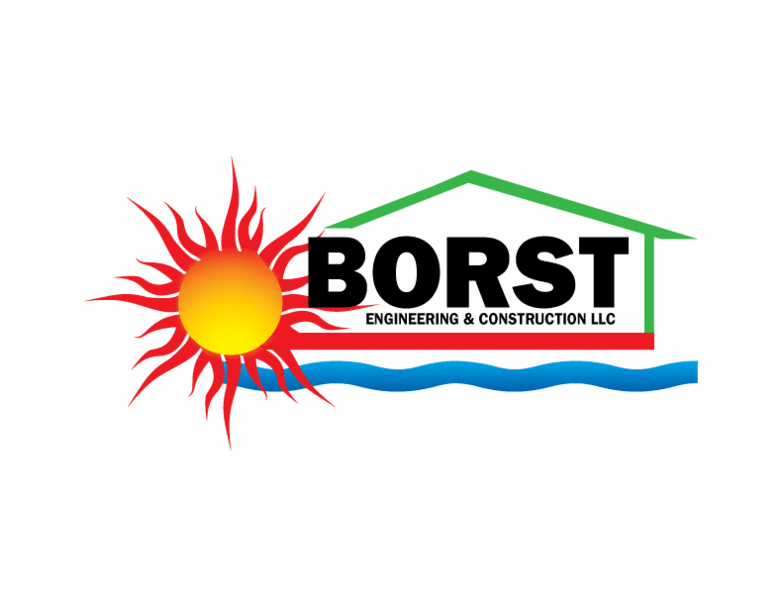Calculator Instructions
Planimeter - Borst Engineering & Construction LLC
 Point 1 Latitude (Deg, South is Negative) Point 1 Longitude (Deg, West is Negative) Point 2 Latitude (Deg, South is Negative) Point 2 Longitude (Deg, West is Negative) Point 3 Latitude (Deg, South is Negative) Point 3 Longitude (Deg, West is Negative) Point 4 Latitude (Deg, South is Negative) Point 4 Longitude (Deg, West is Negative) Point 5 Latitude (Deg, South is Negative) Point 5 Longitude (Deg, West is Negative) Point 6 Latitude (Deg, South is Negative) Point 6 Longitude (Deg, West is Negative) Point 7 Latitude (Deg, South is Negative) Point 7 Longitude (Deg, West is Negative) Point 8 Latitude (Deg, South is Negative) Point 8 Longitude (Deg, West is Negative) Point 9 Latitude (Deg, South is Negative) Point 9 Longitude (Deg, West is Negative) Point 10 Latitude (Deg, South is Negative) Point 10 Longitude (Deg, West is Negative) Point 11 Latitude (Deg, South is Negative) Point 11 Longitude (Deg, West is Negative) Point 12 Latitude (Deg, South is Negative) Point 12 Longitude (Deg, West is Negative) Spherical or Oblate Spheroid Earth (0=Spherical, 1=Oblate)
 Perimeter (Feet) Perimeter (Statute Miles) Area (Square Feet) Area (Acres) Area (Square Statute Miles) Length of Degree of Latitude (Statute Miles) Length of Degree of Longitude (Statute Miles)
Borst Engineering & Construction LLC